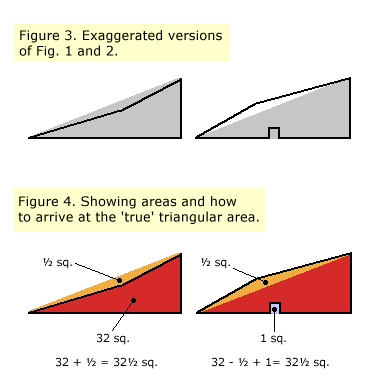## Sunday, February 22, 2015

Consider the diagram below. Figure 1 shows four shapes. The same four shapes have been rearranged in Figure 2. However, there is now a gap, but none of the shapes have changed.How can this be?

You will have tried to calculate the areas of everything involved. The red triangle has an area of 12 squares, the blue triangle has 5 squares, the orange shape has 7, and the green shape has 8. The sum of all these parts is 12 + 5 + 7 + 8 = 32 squares.

You might have thought that the large, enclosing figure was (5 × 13) / 2 = 32.5, and wondered how there is a 0.5 square discrepency.

However, this is not the case. The enclosing figures are not actually a triangles at all: the blue triangle has a gradient of 2 / 5 = 0.4, and the red triangle has a gradient of 3 / 8 = 0.375. The thick black lines aid in deceiving the eye into seeing the 'hypotenuse' as a straight line.Figure 3 shows the differing gradients with exaggerated shapes. Figure 4 shows how Figure 2's "bulge" in its hypotenuse made space for the hole.

OK, so it's a bit of a trick. But you may note that I never said those big "triangles" were actually triangles!